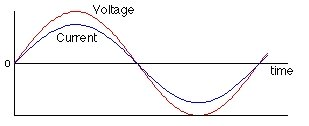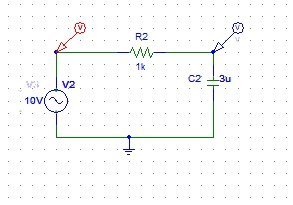# AC circuits analysis in PSpice : tutorial 6

In this tutorial, I will help you do the analysis of an AC circuit. An AC circuit is the one in which the source of the circuit is of alternating current rather than DC (Direct current). At the start of the tutorial a brief introduction of AC (alternating current) is provided after that a simple AC circuit is implemented in PSPICE and the results were analyzed precisely. At the end of the tutorial you are provided with an exercise to do it by yourself, and in the next tutorials I will assume that you have done those exercises and I will not explain the concept regarding them

### Introduction to AC circuits analysis

A simple AC circuit is a circuit in which alternating current source is used. In AC circuit the source oscillates continuously as in the case of a sine wave. A simple AC voltage source is shown in the figure below,Figure 1: Voltage source

On complete cycle of an AC voltage source consists of two half cycles, one being the positive half cycle and the other id the negative half cycle. The polarities in both the half cycles will be different from each other. The same ohms’ law as discussed in the previous tutorial applies on the AC voltage also, the presence of a resistor changes the value of the current accordingly with the resistor if the voltage is kept constant.

### Example of AC circuits Analysis in PSpice

Figure 2: Open new schematic

Figure 3: Saving schematic

Figure 4: Get new part

Figure 5: Inserting a resistor

Figure 6: Capacitor placement

Figure 7: Place input sin

Figure 8: Placing ground

Figure 9: Placed components

Figure 10: Drawing wire

Figure 11: Complete circuit diagram

Figure 12: AC attributes

Figure 13: Capacitor values

Figure 14: Voltage marker

Figure 15: Placed voltage marker

Figure 16: Simulation setup

Figure 17: AC sweep properties

Figure 18: Labeling the wire

Figure 19: Simulation

Figure 20: Output voltage at the capacitor node

• The decreasing sweep at the capacitor node here implies the discharging of the capacitor. As in case of an AC circuit the input signal source consists of two half part or cycles. One is the positive half cycle of the AC signal and the other is the negative half cycle. During the positive half cycle, the direction of the current i.e. the polarity of the voltage will be in accordance with polarity of the capacitor and the capacitor will start charging during the positive half cycle, whereas during the negative half cycle due do the inversion the polarity of the voltage source, the polarity of the capacitor will be opposite to that of the source, hence taking into account the charging and discharging concept of a capacitor, the capacitor will start discharging the stored charge ( during the positive half cycle) to the circuit. The exponentially decreasing curve in the above graph implies the discharging phenomenon of the capacitor.
• Connect another voltage marker at the input of the AC source to the see the AC sweep of the source along with that of the capacitor as shown in the figure below,Figure 21: Marker at input

Figure 22: Output displayed along with input

• The green line at the top represents the input AC sweep of the AC source connect. The straight line represents that the input source is constant (neither increasing nor decreasing with time) unlike that of the capacitor voltage.

Exercise:

• Do the AC analysis of the circuit containing an inductor at the place of capacitor.

<<Previous tutorial                           Next tutorial>>